Binary Operations

Chapter 1 Class 12 Relation and Functions
Serial order wise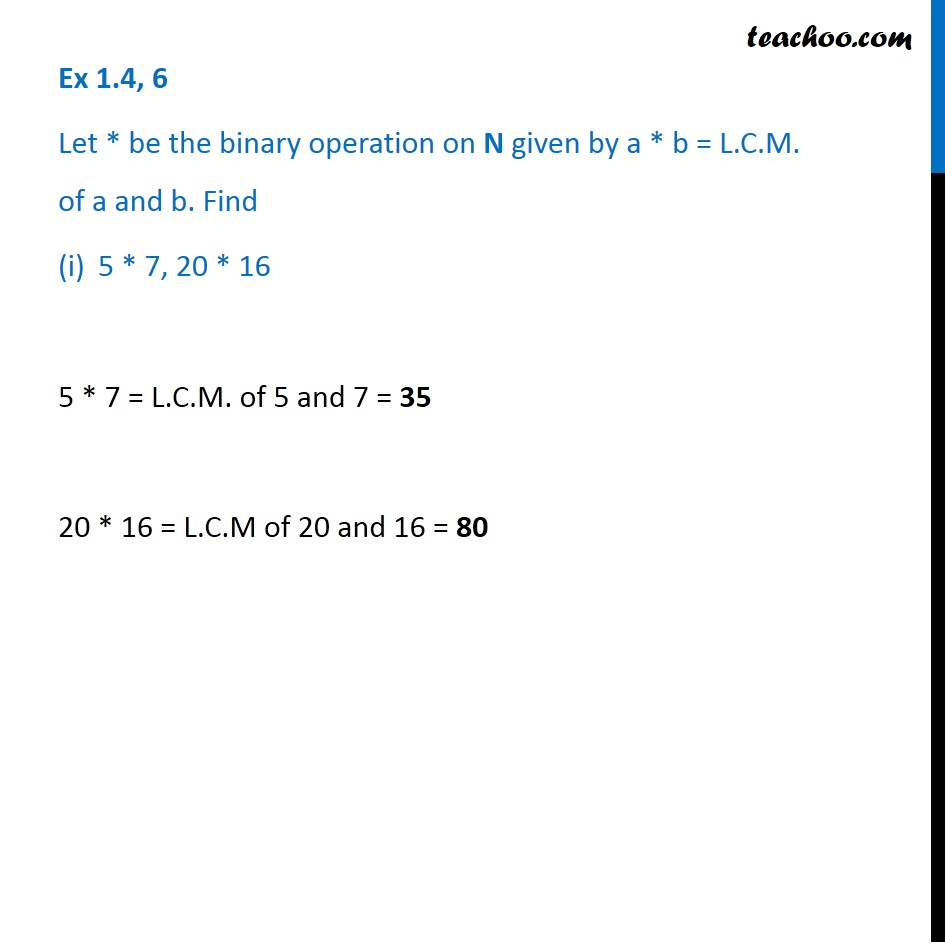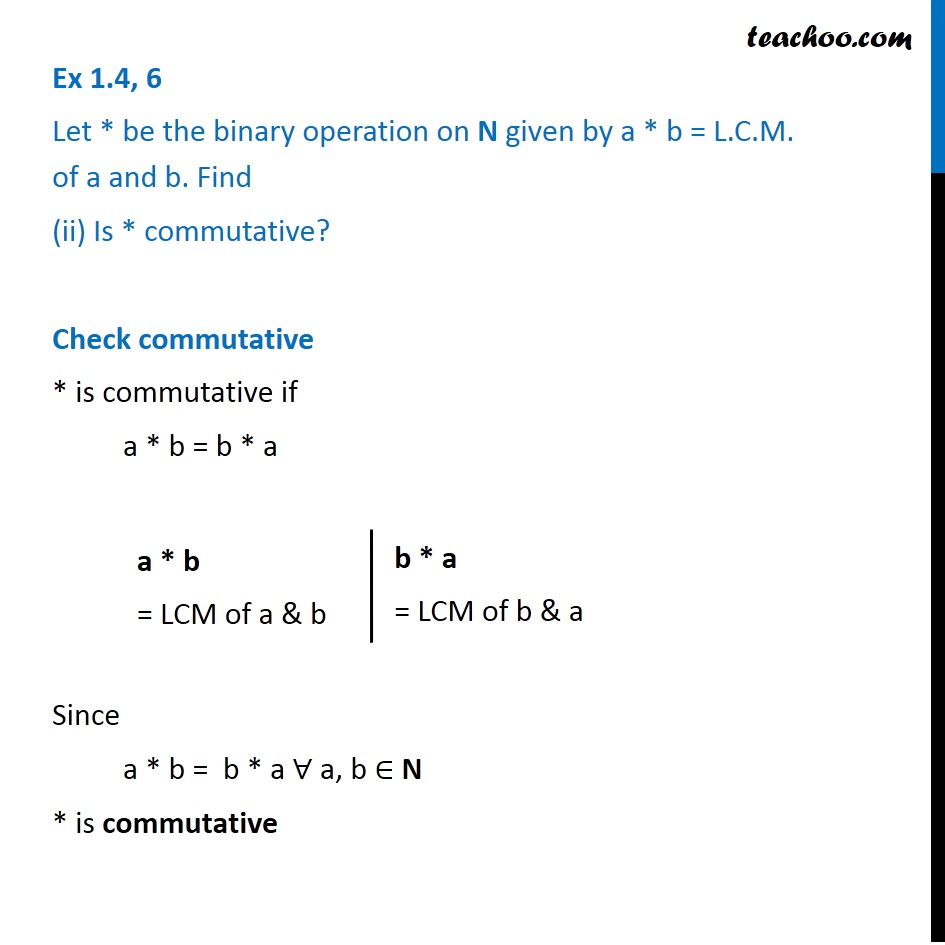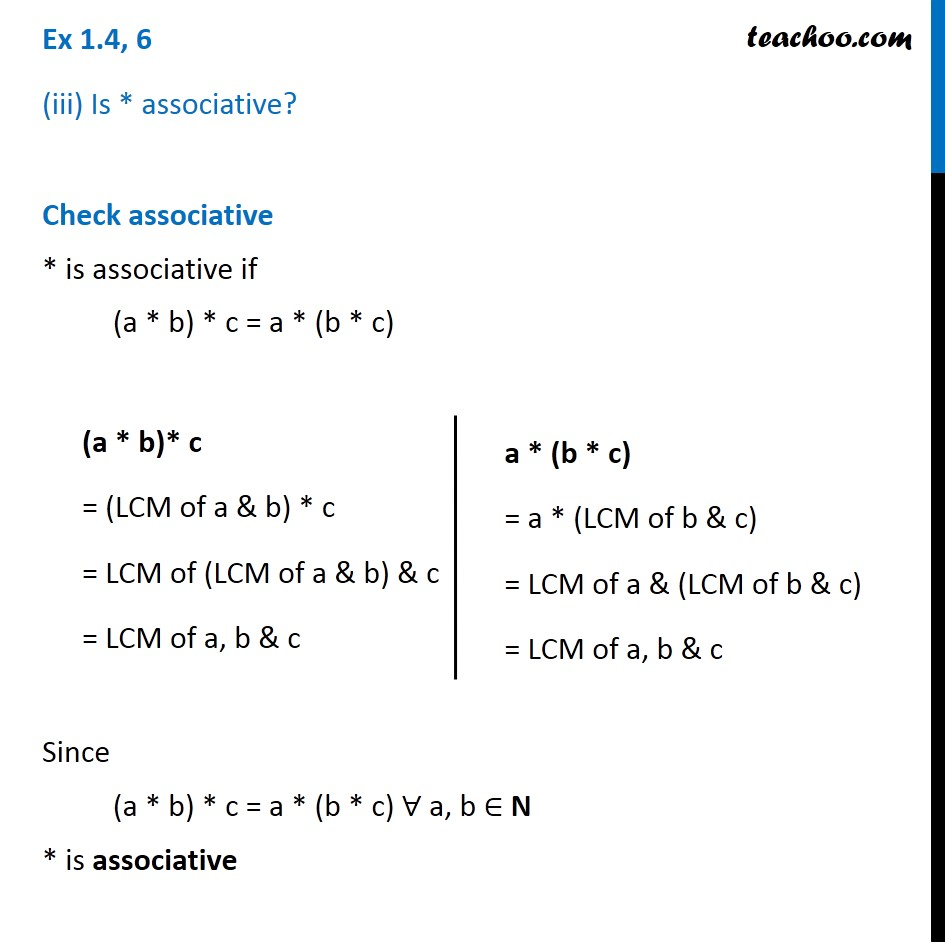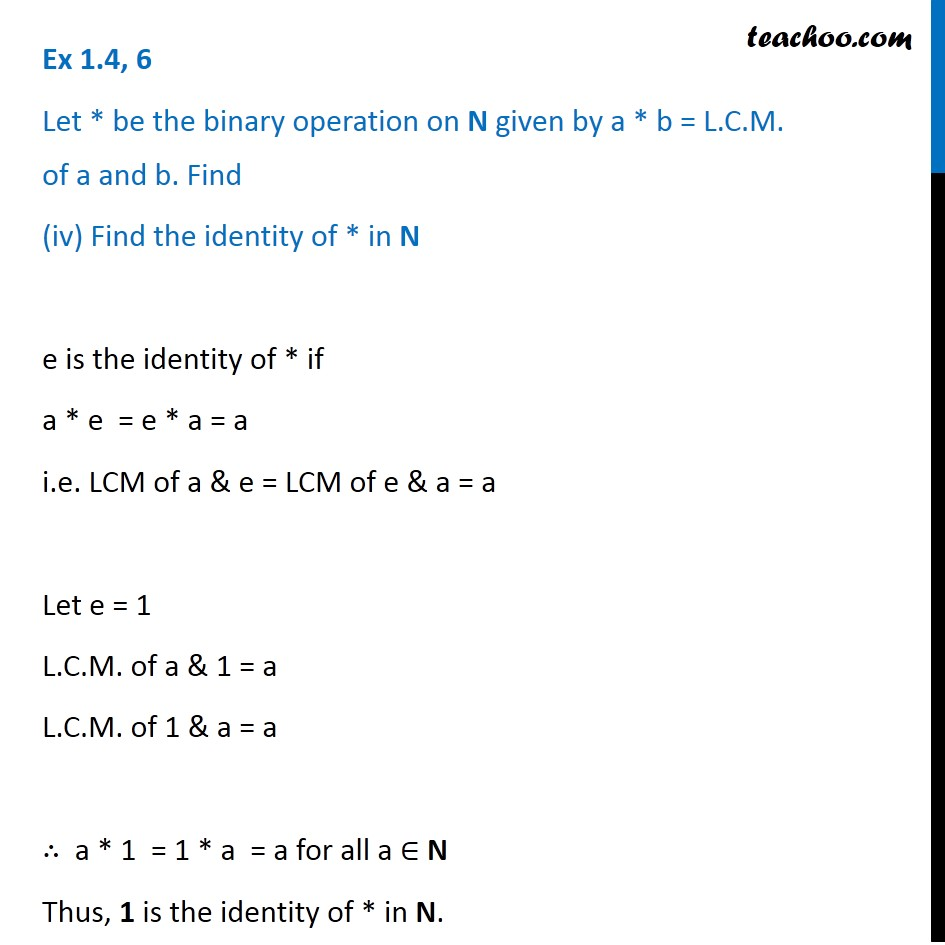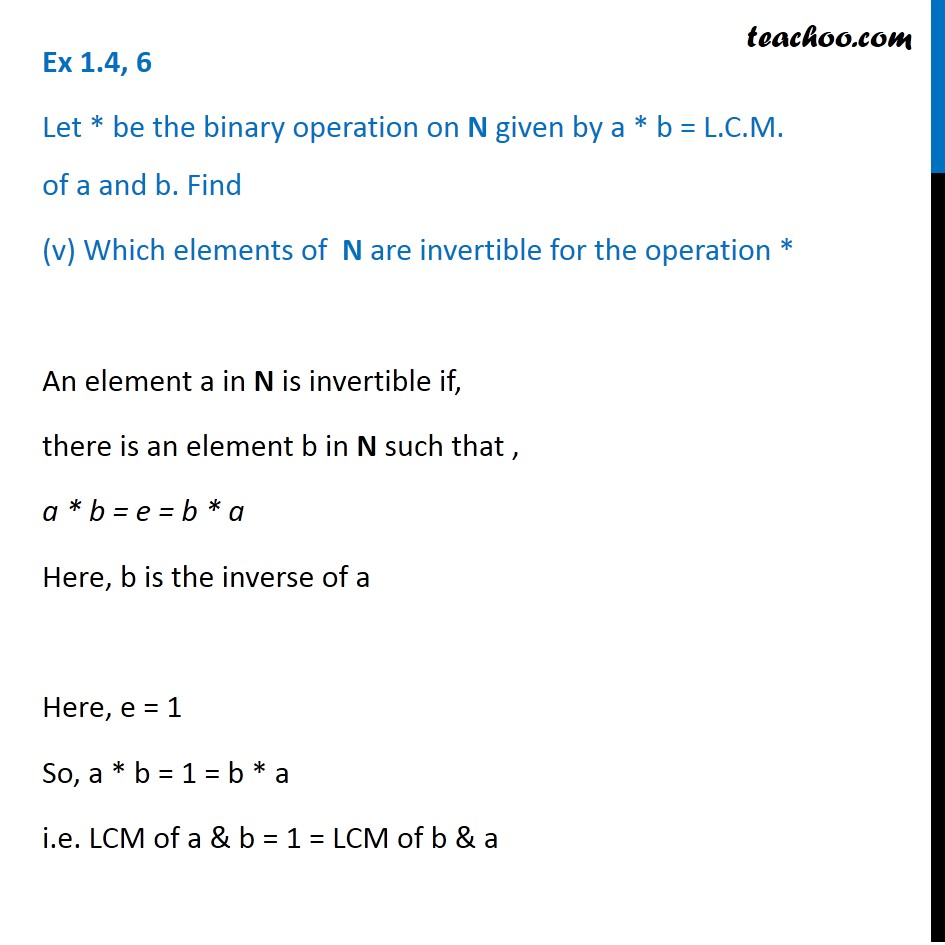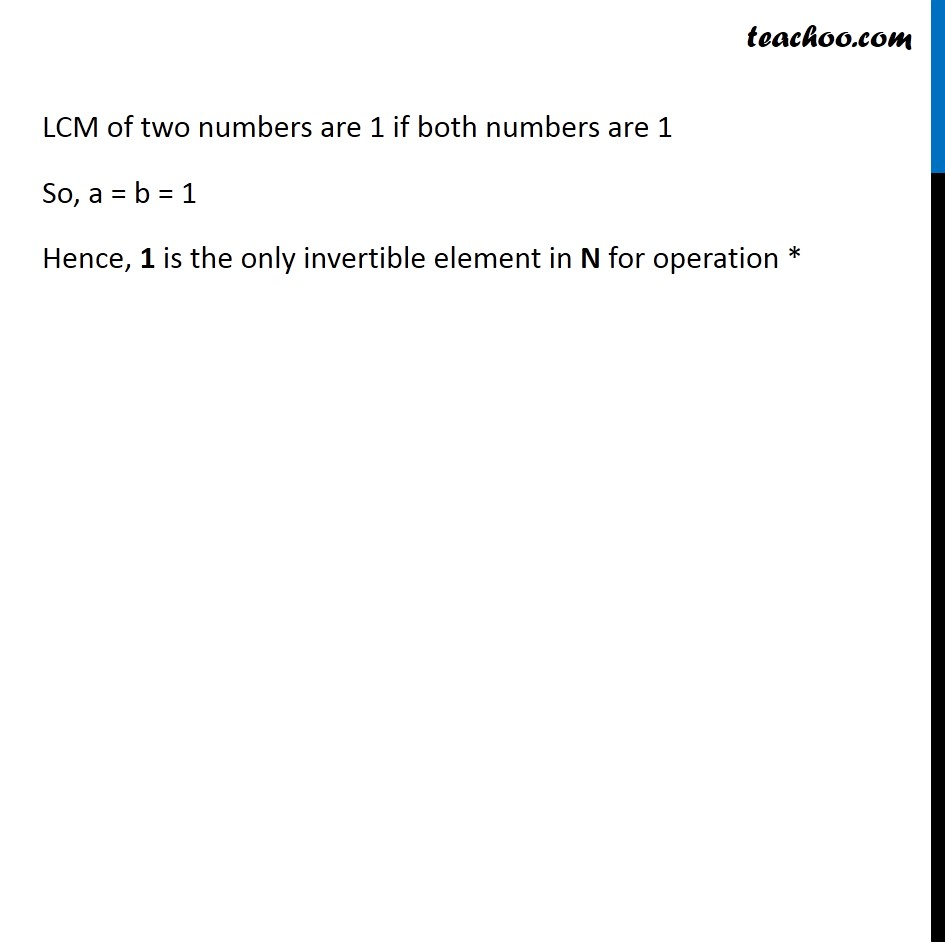Learn in your speed, with individual attention - Teachoo Maths 1-on-1 Class

### Transcript

Ex 1.4, 6 Let * be the binary operation on N given by a * b = L.C.M. of a and b. Find 5 * 7, 20 * 16 5 * 7 = L.C.M. of 5 and 7 = 35 20 * 16 = L.C.M of 20 and 16 = 80 Ex 1.4, 6 Let * be the binary operation on N given by a * b = L.C.M. of a and b. Find (ii) Is * commutative? Check commutative * is commutative if a * b = b * a Since a * b = b * a ∀ a, b ∈ N * is commutative a * b = LCM of a & b b * a = LCM of b & a Ex 1.4, 6 (iii) Is * associative? Check associative * is associative if (a * b) * c = a * (b * c) Since (a * b) * c = a * (b * c) ∀ a, b ∈ N * is associative (a * b)* c = (LCM of a & b) * c = LCM of (LCM of a & b) & c = LCM of a, b & c a * (b * c) = a * (LCM of b & c) = LCM of a & (LCM of b & c) = LCM of a, b & c Ex 1.4, 6 Let * be the binary operation on N given by a * b = L.C.M. of a and b. Find (iv) Find the identity of * in N e is the identity of * if a * e = e * a = a i.e. LCM of a & e = LCM of e & a = a Let e = 1 L.C.M. of a & 1 = a L.C.M. of 1 & a = a ∴ a * 1 = 1 * a = a for all a ∈ N Thus, 1 is the identity of * in N. Ex 1.4, 6 Let * be the binary operation on N given by a * b = L.C.M. of a and b. Find (v) Which elements of N are invertible for the operation * An element a in N is invertible if, there is an element b in N such that , a * b = e = b * a Here, b is the inverse of a Here, e = 1 So, a * b = 1 = b * a i.e. LCM of a & b = 1 = LCM of b & a LCM of two numbers are 1 if both numbers are 1 So, a = b = 1 Hence, 1 is the only invertible element in N for operation *